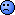## Sample Size II [Power / Sample Size]

Hi All,

Let's say I want to calculate sample size based on results of a previous study.

I have the following data:
n=20
CV=0.18 (for Cmax and AUC)GMR1 = 0.97
GMR2=1.19

If to calculate size based on GMR1 I think it's better to use a script:

sampleN.TOST(CV=0.18, theta0=0.95) +++++++++++ Equivalence test - TOST +++++++++++             Sample size estimation ----------------------------------------------- Study design:  2x2 crossover log-transformed data (multiplicative model) alpha = 0.05, target power = 0.8 BE margins = 0.8 ... 1.25 True ratio = 0.95,  CV = 0.18 Sample size (total)  n     power 16   0.820357if to use GMR = 1.19 we see something terrible:

sampleN.TOST(CV=0.18, theta0=1.19) +++++++++++ Equivalence test - TOST +++++++++++             Sample size estimation ----------------------------------------------- Study design:  2x2 crossover log-transformed data (multiplicative model) alpha = 0.05, target power = 0.8 BE margins = 0.8 ... 1.25 True ratio = 1.19,  CV = 0.18 Sample size (total)  n     power 166   0.803570 - too many people...What to do in such situations?Ing. Helmut Schütz